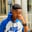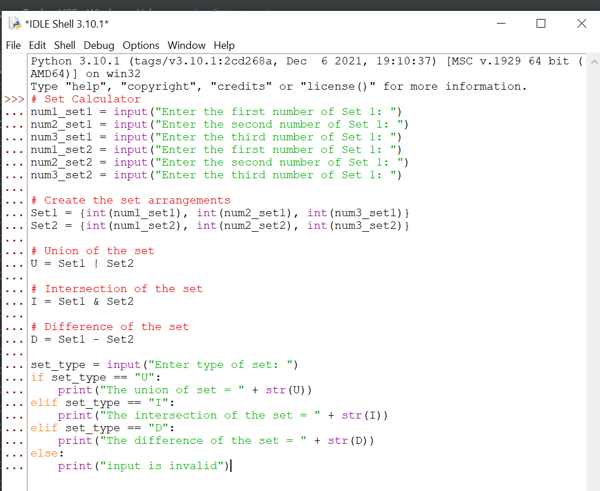Related Tags

python
set
programming
communitycreator

# How to create a set calculator using PythonPeters Ledum Favour

### Introduction

This shot discusses sets, set types, creating programs for some set types, and creating a relatable set calculator. These concepts help programmers understand how Python can easily solve mathematical problems.

### Set

A set can be defined as the collection of elements. Elements can be numbers, places, symbols, etc. A set with no element is classified as a null set.

example: {a, b, c, d, e}

In Python, we use curly brackets {} to represent sets.

### The union of a set

The union of two sets, Set A and Set B, is a set consisting of elements in both Set A and Set B. The union of a set can be defined as the combination of all elements without the repetition of any element. In Python, the union of a set is calculated by the “|” operator. It combines elements without repetition. Let’s take a look at an example:

# Union of a set
Set_A = {2, 4, 7}
Set_B = {3, 2, 9}
print (Set_A | Set_B)

Note: # represents a comment in Python.

### The intersection of a set

The intersection of set is a set consisting of elements that are in set A and set B. If set A and set B have an element in common, it is said that those elements are intersected. The intersection of a set are elements that were repeated in other sets. Here’s an example:

# Intersection of a set
Set_A = {2, 4, 7}
Set_B = {3, 2, 9}
print (Set_A & Set_B)

In the example above, we notice that the “&” operator is used. In Python, the “&” operator gets the intersection of a set. When used appropriately, Python checks for repeated elements and outputs it.

### The difference of a set

The difference of two sets (written as set A\set B or set A - set B) is the set of all elements of set A that are not in set B. The difference of a set is simply removing a particular set’s elements from another set. For example, in set A - set B, set B elements will be removed from set A while the remaining elements will be the result. The following is an example:

# Difference of a Set
Set_A = {2, 4, 7}
Set_B = {3, 2, 9}
print(Set_A - Set_B)

The “-” operator calculates the difference of a set.

### The power of a set

The power of a set can be defined as the number of possible outcomes of a set. The outcomes also include the set itself and an empty set. Therefore, a set’s power is a combination of all subsets of a set, the set itself, and a null set. The general formula is 2^n (2 raised to power n). n represents the number of elements in the given set.

For example, if set A = {a, b, c, d, e}, the number of elements present is 5. We use the general formula 2^n to find the power of a set.

From the question n = 5, we have 2^5 which gives us 32 possible outcomes.

### Creating a Python program to calculate sets

Following is a Python program that helps calculate some types of sets. Here, the user will be asked to enter a certain number of elements and choose the type of set to be calculated. These sets include the union of a set, the intersection of a set, and the difference of a set:

# Set Calculator

# Create the set arrangements
Set1 = {2, 3, 7}
Set2 = {3, 6, 9}

# Union of the set
Union = Set1 | Set2

# Intersection of the set
Intersection = Set1 & Set2

# Difference of the set
Difference = Set1 - Set2
print("The union of set = " + str(Union))
print("The intersection of the set = " + str(Intersection))
print("The difference of the set = " + str(Difference))

The program above is an illustration of how the set calculator should work. The user input is not included. However, the image below shows the source code involving the user input:RELATED TAGS

python
set
programming
communitycreator

CONTRIBUTORPeters Ledum Favour
RELATED COURSES

View all Courses

Keep Exploring

Learn in-demand tech skills in half the time